# SURFACE AREA OF A CUBOID AND A CUBE

## LATERAL SURFACE AREA OF CUBOID:

=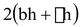Where l = length of cuboid

h = height of cuboid

### TOTAL SURFACE AREA OF CUBE:

Since cube is a cuboid in which length (l) = breadth (b) = height (h) side of cube (a) i.e. l = b = h = a

⇒ Total surface area of cube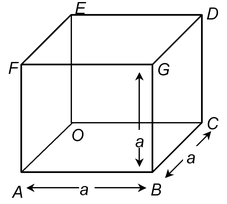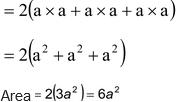### LATERAL SURFACE AREA OF CUBE:

Area =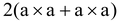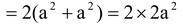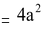So, lateral surface area of cubeWhere a = length of a side.

### LENGTH OF DIAGONAL OF A CUBOID:

Length of diagonal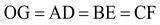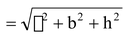l = length; b = breadth; h = height

### LENGTH OF DIAGONAL OF A CUBE

Length of diagonal =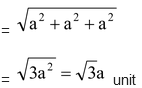Where a = length of a side.